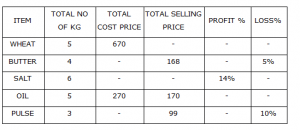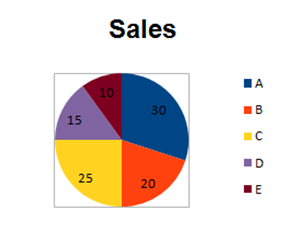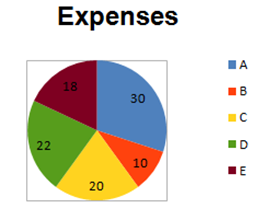## Data Interpretation for SBI PO : Set 03

Direction.1-5) Study the following chart carefully and answer the questions below:1)  If the % of profit on wheat is 12% what is its selling price per kg?

a) 150.08

b) 151.02

c) 162.78

d) 152.92

e) 150.62

a) 150.08

per kg of wheat=670/5=134

100% = 134

112% = x

x=  (134×112)/100=150

2)  What is the difference between the cost price of butter and pulse per kg?

a) 2.5

b) 50

c) 3.34

d) 6

e) 7.54

e) 7.54

Butter:

Selling price (Sp)=168

Cost price (Cp) =(168×100)/95= 176.8

Cp of butter per kg=  176.8/4= 44.21

Pulse:

Selling price  = 99

Cost price =(100×99)/90  = 110

Cp of pulse per kg=  110/3= 36.67

Difference = 44.21-36.67= 7.54

3)  What is the selling percentage of oil? (approximation)

a) 101

b) 69

c) 65

d) 41

e) 63

e) 63

Cp = 270

Sp % =  (100×170)/270=62.96

4)  The average cost price of wheat, Butter and oil per kg?

a) 72

b) 61

c) 78

d) 77

e) 84

d) 77

Per kg of wheat =  670/5= 134

Per Kg of butter =  176/4= 44

Per kg of oil =  270/5 = 54

Average cost =(134+44+54)/3=77

5)  If selling price of salt is Rs.57 per kg. What is total cost price of salt?

a) 400

b) 300

c) 560

d) 310

e) 350

b) 300

Sp of salt = 56 × 6= 342

Sp% => 114% = 342

Cp% => 100% =   (342×100)/114=300

D.6-10) The following pie charts give the value of the sales and expenses of five companies—A,B,C,D and E — as a percentage of the total sales and expenses of these five companies put together.Note:-

I) Profit= sales – Expenses

II) Profit % = (profit/expenses) x 100

III) No company made a loss

6) The ratio of expenses to profit the is highest for which of the following companies? (Consider sales =10lakhs and expense=7.75 lakhs)

a) A

b) B

c) C

d) D

e) E

a) A

Sales

A=(30×10)/100=3lakh

B=(20×10)/100= 2lakh

C=(25×10)/100= 2.5 lakh

D=(15×10)/100=1.5 lakh

E=(10×10)/100=  1lakh

Expense:

A=(7.75×30)/100= 2.325 lakh

B=(7.75×10)/100=0.775lakh

C=(7.75×20)/100= 1.55 lakh

D=(7.75×22)/100= 1.7 lakh

E=(7.75×30)/100=2.325lakh

Profit of A= 3-2.325

= 0.675lakh

Similarly,

B= 0.325lakh

C= 0.95 lakh

D= -0.205

E=-0.395

A=  2.325/0.675  = 3.44

B=  0.775/.325  = 0.58

C=  1.55/0.75  = 1.631

7) If the overall profit percentage of all the five companies put together was 50%, then  how many companies had a profit percentage greater than 60%?

a) None

b) Two

c) Four

d) Three

e) One

1. b) Two

Total sale= 100 x and Total expense =100 Y

(100x-100y)×100/100 y=50

(x-y)/y=1/2

x=3/2 y

Now profit For A= 20 ×3y/2- 10y

= 20y

Similarly,

B=35y/2

C=3y/2

D=-3y

E=15y

Profit percentage A—→ (20y/10y)× 100 = 200%

B—→ (35y/20y)× 100 = 87.5%

C—-> (3y/44y) × 100 = 6.81%

D—→ (-3y/18y)× 100 =50/3%

E—-> (15y/30y)×100 = 50%

8) If the total sales were Rs.600 crore and the overall percentage of profit of all the five companies put together was 100%, what were the expense of company D?

a) Rs.66 crore

b) Rs.68 crore

c) Rs.80 crore

d) Rs.88 crore

e) Rs.75 crore

1. a) Rs.66 crore

Sales = 600

profit %=100

Expense =  600/2  =300 cr

D’ s expense = 300 ×  22/100

= 66 crore

9) What is the least profit percentage of company E?(Rounded off to two decimal places)

a) 22.22%

b) 33.335

c) 44.44%

d) 25.25%

e) 45.45%

c) 44.44%

E’ s expense =(18-10)/18×100= 44.44%

10) If the total expenses were Rs.875 crore and overall percentage profit of all the five companies together was 50%, What were the sales of company B?

a) 222.75 cr

b) 230.75 cr

c) 323.5 cr

d) can’t be determined

e) 262.5 cr